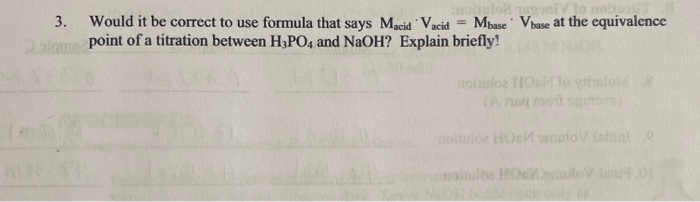# 3. Would it be correct to use formula that says Macid Vacid = Mbase. V base at the equivalence point of a titration...3. Would it be correct to use formula that says Macid Vacid = Mbase. V base at the equivalence point of a titration between H2PO4 and NaOH? Explain briefly!

It would be correct to use formula that says Macid.Vacid=Mbase.Vbase at equivalence point of a titration between H3PO4 and NaOH.

H3PO4 is a weak acid and NaOH is a strong base. And the given formula is valid for the calculation of equivalence point of weak acid and strong base titration.

##### Add Answer of: 3. Would it be correct to use formula that says Macid Vacid = Mbase. V base at the equivalence point of a titration...
More Homework Help Questions Additional questions in this topic.